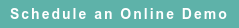# RoyaltyStat Blog

### Posts by Topic

The operating profit margin (measured after depreciation and amortization (OMAD)) of 23,151 companies listed in many countries, reflecting fiscal year-end 2015 accounting results, departs from the usually presumed normal distribution. In this sample, OMAD gets a better fit using the Gamma distribution.

Fig. 1 shows the histogram of OMAD, with a superimposed Gamma function. Fig. 2 shows a probability plot of the same data, and the Gamma function provides a good fit. These figures suggest that the presumed normal distribution underlying the usual calculation of the mean, standard deviation and quartiles is unreliable. This big sample includes companies with a positive OMAD, excluding loss companies.

Since 1989, when we started investigating company-level big data from the IRS SOI, we know that OMAD is skewed, containing long tails with many outliers. This empirical knowledge formed the basis for using quartiles, including the interquartile range, as a method for detecting noncompliance among controlled taxpayers. The extension of the interquartile range as the de facto method to establish an arm's length range of consideration was a regulatory over-simplification, a non sequitur that has become a folly. This questionable assumption about the normal distribution implies also that the computation of industry specific OMAD summary statistics used to tests the reasonableness of judgement selected comparables may be unreliable, unless we consider the frequency distribution of the applicable data.

Blind faith in the normal distribution is not promising. In this case the Gamma distribution reveals that positive OMAD is asymmetric and has a long tail containing many outliers. Instead of calculating the mean of the data as the sum of observations divided by count (N), and the standard deviation as the average deviation from the mean, with denominator N – 1, we must employ the formulae of the Gamma distribution to produce more reliable estimates of the mean and spread or standard deviation. For the Gamma distribution, we can write OMAD ≈ Gamma(α, β), and estimate the α and β parameters using comparables from i = 1 to N observations:

(1)     Mean = α β

(2)     Scale = SQRT(α β2),

where α > 0 is a shape and β > 0 is a scale (average data spread) parameter. These parameter estimates α = 1.171 and β = 11.34 are written on Figs. 1 and 2 below. The Gamma and other relevant distributions for economic applications, such as the lognormal, are detailed in Christian Kleiber & Samuel Kotz, Statistical Size Distribution in Economics and Actuarial Sciences, John Wiley & Sons, 2003, pp. 160-161. K. Krishnamoorthy, Handbook of Statistical Distributions with Applications (2nd edition), Chapman & Hall, 2016, includes the formulae for the computation of confidence and tolerance intervals for many distributions, including the normal, lognormal, and Gamma.

The coefficient of variation is thus:

(3)     CV = 1/SQRT(α), and the mode is

(4)     Mode = β (α – 1) for α > 1, and zero otherwise.

Skewness is equal to 2 / SQRT(α), and thus depends only on the shape parameter.  See also Robert Hogg & Allen Craig, Introduction to Mathematical Statistics (2nd edition), Macmillan, 1965, section 3.3, or Paul Hoel, Introduction to Mathematical Statistics (5th edition), John Wiley & Sons, 1984, section 3.5.

Industry specific OMAD might obey other frequency distributions, such as the lognormal; However, the normal distribution is an unlikely candidate because of pervasive data asymmetry, long tails, and outliers. RoyaltyStat provides subscription based royalty rates, company financials (OMAD), annual reports, and built-in statistical tools—including the estimates of α, β of the Gamma function, and the associated mean, standard deviation, and quartiles. We also compute online summary statistics for several other statistical functions applicable to economic variables, such as royalty rates and OMAD, including the lognormal, Pareto, and Weibull distributions.

The naive attachment to the normal distribution can produce unreliable results because the comparables used to determine arm’s length consideration and ascertain “true taxable income” in transfer pricing are selected using non-random (judgement) procedures. Further, the resulting samples are usually small, containing from eight to 15 observations. Under this double jeopardy (untested assumption of the normal distribution and small samples), the proposed “true taxable income” is vulnerable to attack because falsifiability can be invoked by questioning the factual demonstrability of the underlying data distribution.

The practice of using small samples of selected comparables via bias-prone judgement procedures makes it difficult to ascertain the underlying data distribution. Since there may be no empirical reason to accept the assumption that the normal distribution is valid, heuristic appeals to the law of large numbers are likely to be unconvincing. Thus, empirical challenges to the assumed normal distribution suggest that the arm’s length standard (based on judgement selected comparables) is flawed, and needs to be replaced by more reliable methods. One strong candidate (with scientific admissibility) is to allow industry specific random sampling with censored data. Censoring is important to control the distorting effects of outliers before the computation of the mean and standard deviation of the applicable frequency distribution. We could then be confident that the arm's length range of royalty rates or OMAD would be narrow and resilient to argumentum ad hominem.Published on Oct 21, 2016 7:13:23 AM

Ednaldo Silva (Ph.D.) is founder and managing director of RoyaltyStat. He helped draft the US transfer pricing regulations and developed the comparable profits method called TNNM by the OECD. He can be contacted at: esilva@royaltystat.com

RoyaltyStat provides premier online databases of royalty rates extracted from unredacted license agreements
and normalized company financials (income statement, balance sheet, cash flow). We provide high-quality data, built-in analytical tools, customer training and attentive technical support.Topics: Net Profit Indicator